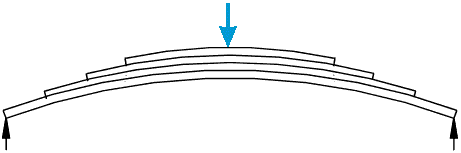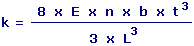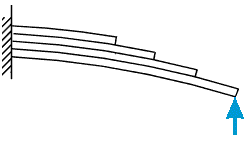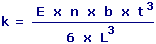Leaf Springs Calculator Enter value and click on calculate. Result will be displayed.

Calculate Stiffness for Semi-Elliptic Laminated Leaf Spring
Calculate Stiffness for Quarter-Elliptic Laminated Leaf Spring

 Web www.calculatoredge.com

Calculate Stiffness for Semi-Elliptic Laminated Leaf SpringE = Youngs Modulus n = Number of Leaves b = Width of Leaves t = Thickness of Leaves L = Span k = Stiffness
 Enter your values: Youngs Modulus (E): Nm-2 Number of Leaves (n): Span(L): m Width of Leaves (b): m Thickness of Leaves (t): m Result: Stiffness (k): Nm-1

Calculate Stiffness for Quarter-Elliptic Laminated Leaf SpringE = Youngs Modulus n = Number of Leaves b = Width of Leaves t = Thickness of Leaves L = Span k = Stiffness
 Enter your values: Youngs Modulus (E): Nm-2 Number of Leaves (n): Span(L): m Width of Leaves (b): m Thickness of Leaves (t): m Result: Stiffness (k): Nm-1LINKSDISCLAIMERCONTACT US